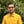Trusted answers to developer questions
Trusted Answers to Developer Questions

Related Tags

d
expm1
communitycreator

# What is the expm1() function in D?Harris Amjad

Grokking Modern System Design Interview for Engineers & Managers

Ace your System Design Interview and take your career to the next level. Learn to handle the design of applications like Netflix, Quora, Facebook, Uber, and many more in a 45-min interview. Learn the RESHADED framework for architecting web-scale applications by determining requirements, constraints, and assumptions before diving into a step-by-step design process.

### Overview

The expm1() function in D returns the mathematical $e$ raised to the power of a specific number minus 1. Here, Euler’s number, $e$, is the base of the natural logarithm.

The mathematical representation of the expm1() function is shown below:

The mathematical representation of the expm1() function

Note: We need to import std.math in our code to use the expm1() function. We can import it using this:
import std.math

### Syntax

expm1(number)
// number should be float, double or real.


### Parameters

This function requires a number as a parameter.

### Return value

This function returns the mathematical $e$ raised to the power of number minus 1 sent as a parameter.

Note:

• If the parameter value is positive infinity, expm1() returns positive infinity.
• If the parameter value is negative infinity, expm1() returns -1.
• If the parameter value is NaN, expm1() returns NaN.
• If the parameter value is -NaN, then expm1() returns -NaN.

### Example

import core.stdc.stdio;import std.stdio;//header required for functionimport std.math;int main(){    //integer    writeln ("The value of expm1(10.0) : ",expm1(10.0));    //positive double value    writeln ("The value of expm1(2.56) : ",expm1(2.56));    //negative double value    writeln ("The value of expm1(-2.56) : ",expm1(-2.56));    //zero    writeln ("The value of expm1(0.0) : ",expm1(0.0));    //exceptional outputs    writeln ("The value of expm1(real.infinity) : ",expm1(real.infinity));    writeln ("The value of expm1(-real.infinity) : ",expm1(-real.infinity));    writeln ("The value of expm1(real.nan) : ",expm1(real.nan));    writeln ("The value of expm1(-real.nan) : ",expm1(-real.nan));    return 0;}

### Explanation

• Line 4: We add the header, std.math, required for the expm1() function.

• Line 9: We use expm1() to calculate the integer value’s exponent minus 1.

• Line 12: We use expm1() to calculate the positive double value’s exponent minus 1.

• Line 15: We use expm1() to calculate the negative double value’s exponent minus 1.

• Line 18: We use expm1() to calculate the zero’s exponent minus 1.

• Line 20–26: We use expm1() to calculate the exceptional numbers’ exponent minus 1.

RELATED TAGS

d
expm1
communitycreator

CONTRIBUTORHarris Amjad

Grokking Modern System Design Interview for Engineers & Managers

Ace your System Design Interview and take your career to the next level. Learn to handle the design of applications like Netflix, Quora, Facebook, Uber, and many more in a 45-min interview. Learn the RESHADED framework for architecting web-scale applications by determining requirements, constraints, and assumptions before diving into a step-by-step design process.

Keep Exploring

Learn in-demand tech skills in half the time